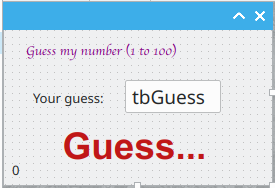# Invariant Binary Search

### Guessing game

Say we were playing a guessing game where I pick an integer between 1 and 100 and you guess it. For each guess, I tell you if its greater than, less than, or equal to my number. How would you play this so that you minimize the number of guesses? The naive approach would be to start from 1 and go upto 100. The worst case here is that if I picked the number 100, you would need 100 guesses. Is there a way to improve?### Binary Search

Say that my number was 43. Using the naive linear search, you would need 43 guesses. However, the "smarter" way to play this game would be to start halfway at 50. Then, I'll tell you that my number is less than 50 so you effectively eliminate half of the possible guesses. Repeating this process, the search sequence would look like this:

50, 25, 37, 43

For our first guess, we knew our number had to be between 1 and a 100 so we guess halfway at 50.

For the second guess, we know it is less than 50, so it is between 1and 49 so we guess 25.

Third guess: lower bound = 26, upper bound = 49 so we guess 37.

Fourth guess: lower bound = 38, upper bound = 49, guess = 43.